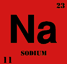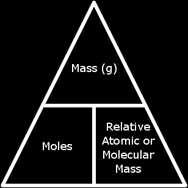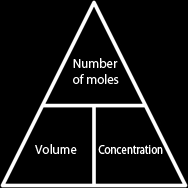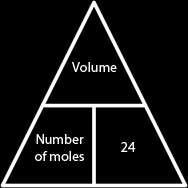# Moles and Empirical Formula

### The Mole Concept:

A mole is a unit to count the number of atoms, ions or molecules. They believed that, for example, if one molecule of carbon dioxide (CO2) contained 1 carbon atom and 2 oxygen atoms, then the ratio of carbon atoms to oxygen atoms is 1:2. So if we wanted to make 100 molecules of carbon dioxide without any excess of the re-actants we will use 200 atoms of oxygen. We got this by:

 1 Carbon 2 Oxygen Amount of carbon atoms = 1 x 100 = 100 Amount of oxygen atoms= 2 x 100 = 200

Chemists use a method similar to that one, but on a larger scale, in industries to prevent wasting money by buying excess substances that will not be used. This is called Avogadro’s Constant.

Avogadro was a scientist in the 19th century. He discovered a relationship between a certain amount of substance (atoms, ions or molecules) and the Ar (Relative atomic mass) or Mr (Relative Molecular Mass) of the substance.

 The Ar of an element is its Mass Number in the periodic table. For example:The Ar  of sodium is 23

The Mr of a compound is the sum of the Ar of all the atoms present in one molecule of the compound.

 The Mr of Carbon dioxide (CO2) is: The Ar of carbon atom + (2 x the Ar of oxygen atom) 12 + (2 x 16) = 44 So the Mr of carbon dioxide is 44

What Avogadro discovered is that if I am holding 6x1023 atoms in my hand, its mass is equal to the Ar of Iron (Fe).
This unit is called Mole.

 6x1023 is not an equation; it is the number of atoms, ions or molecules in one mole. If you put 6x1023 in a calculator, you will find out that this number is 600,000,000,000,000,000,000. So if I am holding in my hands 600,000,000,000,000,000,000 atoms of iron, then I am holding 1 mole of iron. This is 56 grams heavy because the Ar of iron is 56.From this we conclude that the mass of one mole of any substance is the Ar of it (if it was an element) or the Mr of it (if it was a compound). The mass of one mole of any substance is expressed as the molar mass, and the word mole can be abbreviated with mol. The molar mass is always expressed in grams.

 Molar mass of carbon is 12g Molar mass of oxygen is 16g Molar mass of sodium is 23g Molar mass of iron is 56g

The molar mass of a compound is Mr of it:

 The molar mass of an oxygen molecule (O2) is: 2x16= 32g The molar mass of sodium chloride (NaCl) is: 23+35.5= 58.5g The molar mass of sulphuric acid (H2SO4) is: (2x1)+32+ (4x16) = 98g

The mass of 2 moles of a substance is 2x (Ar or Mr), the mass of 3 moles of a substance is 3x (Ar or Mr)..

 The mass of 6 moles of water (H2O) is: Mr of H2O: (2x1) +16= 18 6mol of (H2O) is: 6x18= 108g The mass of 9 moles of hydrated copper sulphate (CuSO4.5H2O) is: Mr of CuSO4.5H2O: 64+32+ (9x16) + (10x1) = 250 9mol of CuSO4.5H2O is: 9x250= 2250g or 2.25kg

If we wanted the mass of a sample of a compound, we had to know its Mr and the numbers of moles of it we have, and multiply both. We can also find the number of moles in a sample of a compound if we know the mass of the sample and the Mr of it.

Remember that the Mr of a substance is how much one mole of it weighs.

 How many moles are in 36 grams of water (H2O)? Mr of water: (2 x 1) + 16 = 18 If one mole of water weighs 18g, then 32g of water must be: Mole = 32 ÷ 18 = 2mol How many moles are there in 4 grams of sodium hydroxide (NaOH)? Mr of NaSO4 = 23 + 16 + 1= 40 Moles = 4 ÷ 40 = 0.1mol

From this we conclude that there is a relation between the mass of a substance, its molar mass, and the number of moles in it.

 Mass of sample = Moles × Molar Mass (Ar or Mr) Moles = Mass of sample ÷ Molar Mass (Ar or Mr) Molar Mass (Ar or Mr) = Mass of sample ÷ MolesSometimes we need to find concentration of a solution. The unit of concentration can be g/dm3 or mol/dm3.

Literally, mol/dm3 means how many moles of the solute are dissolved in every dm3 of the solvent. So if salt and water solution has a concentration of 3 mol/dm3, then in every dm3 of water, there are 3 mols of salt dissolved. This means that in order for us to find the concentration of a solution, we divide the amount of solute (in moles) in the solution by the total volume of the solution.

 Calculate the concentration (mol/dm3) of a solution containing 4 moles of sulphuric acid and has a volume of 2 dm3. Concentration = Moles of solute ÷ Volume of solution Concentration = 4 ÷ 2 = 2 mol/dm3

If we want to find the number of moles dissolved in a solution, we'll need to know both concentration and the volume of the solution.

 Find the number of moles of sulphuric acid dissolved in water if the solution has a concentration 2 mol/dm3 and a volume of 25 dm3. Moles of solute = Concentration x Volume of solution Moles of solute = 2 x 25 = 50 mol of sulphuric acid

We can also find the volume of a solution, if we know the concentration and number of moles of solute dissolved; we divide the number of moles by the concentration.

 Find the volume of a solution containing 4 moles of sulphuric acid with concentration 2 mol/dm3. Volume of solution = Moles of solute ÷ Concentration Volume of solution = 4 ÷ 2 = 2dm3

From this we conclude that the relation between the volume, concentration and number of moles dissolved in a solution is:

 Number of moles = Volume × Concentration Concentration = Number of moles ÷ Volume Volume = Number of moles ÷ ConcentrationIn gases it is a different story to solutions and solids because weighing a gas is very difficult, and we have no concentration. So in gases, we use volume of the gas to find how many moles are in it.

Scientists have proved that any gas, will have a volume of 24 dm3 provided that is it is at room temperature and pressure (R.T.P). That means that all gases at r.t.p occupy 24 dm3. We use this theory to find out how many moles are present in some gas if we have its volume, we just divide it by 24.

 How many moles of carbon dioxide are there, if the gas occupied 72 dm3? We know that every 1 mole occupies 24 dm3, so 72 dm3 are occupied by: Number of moles = Volume of gas ÷ 24 Number of moles = 72 ÷ 24 = 3 mol

We could also find the volume of a gas if we know the number of moles we have in it, we simply multiply it by 24.

 What is volume occupied by nitrogen gas, if 6 moles of it are present? We know that each mole occupies 24dm3 and that we have 6 moles, so they will occupy: Volume = Number of moles x 24 Volume = 6 x 24 = 144dm3

So we conclude that the relation between the number of moles present in a gas and its volume is:

 Volume = Number of moles × 24 Number of moles = Volume ÷ 24### Reactions and Mole Ratio:

What volume of carbon dioxide (CO2) at R.T.P will be produced when 50g of calcium carbonate react with an excess of hydrochloric acid:

 CaCO3 + 2HCl → CaCl2 + H2O + CO2 1 : 2 1 : 1 : 1

First we write the mole ratio of each reactant and product.
Now we find the number of moles in 50g of CaCO3:
Number of moles = Mass ÷ Mr
Number of moles = 50 ÷ 100 = 0.5 mol

If the mole ratio of CaCO3 to CO2 is 1:1, then we must also have 0.5 mol of CO2, if we have 0.5 mol of CO2, then we can get the volume produced:
Volume = Number of moles x 24
Volume = 0.5 x 24 = 12dm3
Volume of CO2 Produced is 12dm3

If all reactants are gases, then the mole ratio is also the volume ratio:

Calculate the volume of methane needed to react with 70 dm3 of oxygen:

 CH4 + 2O2 → CO2 + 2H2O 1 : 2 1 : 2

First we write the mole ratio of all reactants and products.
If both reactants are gases, then the mole ratio is also the volume ratio, that means if we have 70 dm3 of O2 and the ratio of O2 to CH4 is 2:1, then the volume of CH4 is half the volume of O2:

0.5 x 70 = 35

Volume of methane needed is 35 dm3.

Note: The total mass of the reactants must always equal the total mass of the products.

200g of pure calcium carbonate decomposes to calcium oxide and carbon dioxide. Calculate the mass of CaO produced and the volume of CO2 produced at R.T.P.:

 CaCO3 → CaO + CO2 1 1 : 1

First we write the mole ratio of the reactant and the products.
Mr of CaCO3 is 40 + 12 + (3 x 16) = 100; moles of CaCO3= 200 ÷ 100 = 2 mols
Then we have 2 mols of CaO, because the mole ratio is 1:1, mass of CaO = 2 x 56 = 112g of CaO is produced.
And if we have 2mols of CO2, because the ratio is 1:1, then the volume of CO2 produced is: 2 x 24 = 48.
48 dm3 of CO2 is produced.

### Percentage Purity:

If we have a sample of reactant that is not pure, we can find how pure it is by finding the mass of it that reacted. The impurities are assumed to not interfere with the reaction. Then we divide the mass that reacted by the total mass and multiply it by 100 to get the percentage.

 Percentage Purity = Pure mass x 100 Total mass

When 10g of impure zinc reacted with dilute sulphuric acid, 2.4 dm3 of hydrogen gas were collected at R.T.P. Calculate the percentage purity of zinc:

 Zn + H2SO4 → ZnSO4 + H2 1 : 1 1 : 1

First we have to find the number of moles in any of the chemicals in the reaction to find the number of moles of zinc that reacted. We know that we 2.4 dm3 of hydrogen are produced, we can find how many moles this is by:
Number of moles = Volume ÷ 24
Number of moles = 2.4 ÷ 24 = 0.1 mol

If we have 0.1 mol of hydrogen and the mole ratio of hydrogen to zinc is 1:1 then we must also have 0.1 mol of zinc. Now we have to find how much 0.1 mol of zinc weigh:
Mass = Moles x Ar
Mass = 0.1 x 65 = 6.5g

If 6.5g of zinc are present in the sample, then the percentage purity is:
% Purity = (Pure mass ÷ Total mass) x 100
% Purity = (6.5 ÷ 10) x 100 = 65%

### Percentage Yield:

Percentage yield is the mass of a substance produced in a reaction as a percentage of the calculated mass. That means that in a reaction, the calculations showed that the 50 grams of calcium oxide will be produced, but practically, only 45 grams were produced then the percentage yield is 45 divided by 50 multiplied by 100, which is 90%:

 Percentage yield = Mass produced x 100 Mass predicted

Heating 12.4g of Copper (II) Carbonate Produced only 7g of Copper (II) Oxide. Find the percentage yield of Copper (II) Oxide:

 CuCO3 → CuO + CO2 1 1 : 1

First we calculate the mass of CuO that is supposed to be produced:
We write the mole ratio of the reactant and the products.

Mr of CuCO3 is 124 we have 12.4g so the number of moles is 12.4 ÷ 124 = 0.1; if the ratio of CuCO3 to CuO is 1:1, then we must also have 0.1 mol of CuO, the Mr of CuO is 80. Then the mass of CuO must be 0.1 x 80 = 8g. We actually got 7g so the percentage yield is:

Percentage yield = (Mass produced ÷ Mass predicted) x 100
Percentage yield = (7 ÷ 8) x 100 = 87.5%
So the percentage yield is 87.5%

### Composition Percentage of Elements in Compounds:

This is a way to find the percentage of an element in a whole compound. For example, if we have the compound CaCO3, we can find the percentage of any of the elements in it by the following rule:

 Composition percentage = Number of atoms of the element in a molecule x Ar x 100 Mr of the compound

 Find the percentage of nitrogen in the following compounds: Ammonium Nitrate, NH4NO3: Ammonium Sulphate, (NH4)2SO4: Urea, CO(NH2)2: Answers: [(14 x 2) ÷ 80] x 100 = 35% [(14 x 2) ÷ 132] x 100 = 21.21% [(2 x 14) ÷ 60] x 100 = 46.6%

### The Empirical Formula:

The molecular formula shows the actual number of atoms of each element in a compound, but the empirical formula is a formula that shows the simplest ratio of atoms present in a compound. For example if a compound has the molecular formula C4H8, its empirical formula would be(CH2)n, n is the number to multiply by to get the molecular formula, which is 4 in this case, the 8 is divided by the 4 to give the simplest ratio between them. The empirical formula is widely used with hydrocarbons which are compounds containing hydrogen and carbon, and carbohydrates, which are compounds containing carbon and water.

A carbohydrate has 40% of its mass carbon, 6.66% hydrogen. Find the compound’s empirical and molecular formula given that is Mr is 180:

We assume that we’ve got 100g of this carbohydrate. Then we have 40g of carbon and 6.66g of hydrogen, we can now find oxygen’s mass and the number of moles we have of each element, thus we can get the simplest ratio between them and get the empirical formula.

Carbon Hydrogen Oxygen
Mass % 40 6.66 100 - 46.6 = 53.34
Ar 12 1 16
Moles (40 ÷ 12) = 3.33 (6.66 ÷ 1) = 6.66 (53.34 ÷ 16) = 3.33
Simple ratio 1 2 1
Empirical formula C H2 O

Emperical formula: CH2O

N = Molecular Mr ÷ Empirical Mr
N = 180 ÷ 30 = 6

Molecular Formula = C6H12O6